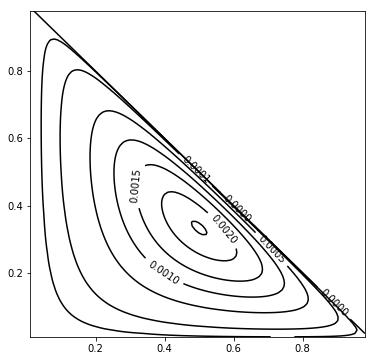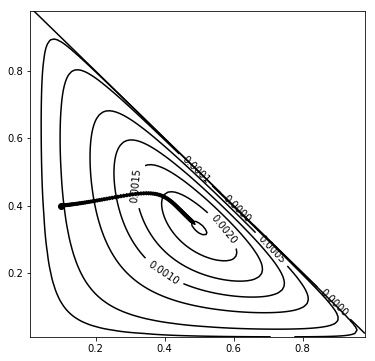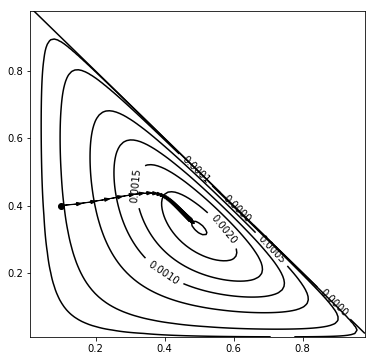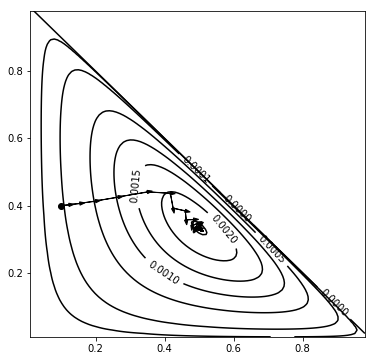# Maximum likelihood estimation and numerical optimization¶

In :
import autograd.numpy as np
np.seterr(all="ignore")
import matplotlib.pyplot as plt
%matplotlib inline


Suppose we want to estimate the probability of a coin landing heads (H), tails (T), or on the edge (E):

In :
data = "HTTHEH"
ch = sum([1 for c in data if c == "H"])
ct = sum([1 for c in data if c == "T"])
ce = sum([1 for c in data if c == "E"])
print(ch, ct, ce)

3 2 1


Our model has two parameters, $\theta_H$ and $\theta_T$, the probability of heads and tails, respectively. In terms of these two parameters, the probability of seeing the data that we saw would be:

In :
def likelihood(theta):
ph, pt = theta
pe = 1-ph-pt
return ph**ch * pt**ct * pe**ce
eps = 1e-2
X, Y = np.meshgrid(np.arange(eps, 1-eps, 0.01), np.arange(eps, 1-eps, 0.01))

def plot():
fig = plt.figure(figsize=(6,6))
CS = plt.contour(X, Y, likelihood((X, Y)), [0,1e-5,1e-4,5e-4,1e-3,1.5e-3,2e-3,2.3e-3], colors='k')
plt.clabel(CS, inline=1, fontsize=10, fmt="%1.4f")

plot()Below, we're going to make use of the gradient (vector of first derivatives) of the function, which we compute using a magic package called Autograd:

In :
g = ag.grad(likelihood)


In stochastic gradient ascent/descent, at each step, we move a little bit in the direction that the surface rises/falls most steeply. The step size or learning rate $\eta$ controls how quickly we move. If it is too high, then we can overshoot, as seen below.

In :
def plot_path(path):
plot()
x0, y0 = path
plt.plot(x0, y0, marker='o', color='k')
for xy1 in path[1:]:
x1, y1 = xy1
plt.arrow(x0, y0, x1-x0, y1-y0, head_width=0.01, color='k')
x0, y0 = x1, y1

theta0 = np.array([0.1, 0.4])
theta = theta0
path = [theta]
eta = 1
for iteration in range(100):
theta1 = theta + eta*g(theta)
path.append(theta1)
theta = theta1
plot_path(path)One way to fix this is to make the step size decrease over time. But be careful not to decrease it too much, or convergence may take a long time.

In :
theta = theta0
path = [theta]
eta = 20
for iteration in range(100):
theta1 = theta + eta/(iteration+1)*g(theta)
path.append(theta1)
theta = theta1
plot_path(path)Another trick is to halve the learning rate when the objective function doesn't improve.

In :
theta = theta0
path = [theta]
eta = 10
for iteration in range(100):
theta1 = theta + eta*g(theta)
path.append(theta1)
if likelihood(theta1) <= likelihood(theta): eta /= 2
theta = theta1
plot_path(path)